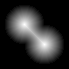## 二值图像的距离变换

`bwdist` 函数支持多种距离度量。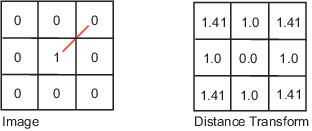City block

City block 距离度量基于 4 连通邻域来测量像素之间的路径。边缘接触的像素相隔 1 个单位；以对角方式接触的像素相隔 2 个单位。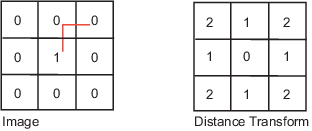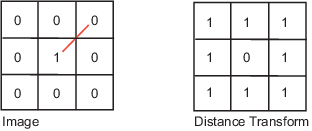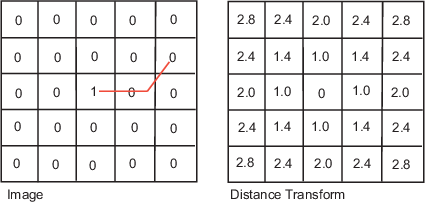```center1 = -10; center2 = -center1; dist = sqrt(2*(2*center1)^2); radius = dist/2 * 1.4; lims = [floor(center1-1.2*radius) ceil(center2+1.2*radius)]; [x,y] = meshgrid(lims(1):lims(2)); bw1 = sqrt((x-center1).^2 + (y-center1).^2) <= radius; bw2 = sqrt((x-center2).^2 + (y-center2).^2) <= radius; bw = bw1 | bw2; figure imshow(bw)```

```D = bwdist(~bw); figure imshow(D,[])```